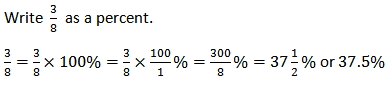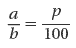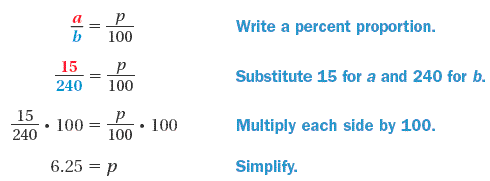Understanding Percent

Understanding Percent

Writing Fractions or Decimals as Percents

A fraction or decimal can be rewritten as a percent if you multiply it by 100%.

Example

Rewrite a fraction as a percent.Example

Rewrite a decimal as a percent.

Solve Percentage Problems by Using Proportion

The word percent means "per hundred." A percent is a ratio with a denominator of 100. The symbol for percent is %.

To represent "a is p percent of b," use proportion:where a is part of the base b, and p is the percentage.

Example

The city council decides to plant trees as part of an erosion prevention project. Out of 240 newly planted trees, 15 are oak. What percentage of the trees are oak?

Solution

To find the percentage of trees that are oak, use a percent proportion.6.25% of the newly planted trees are oak.